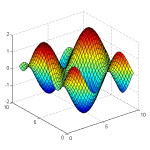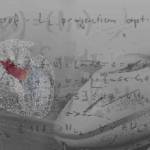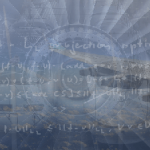# Computational Mathematics courses

Computational mathematics involves mathematical research in areas of science where computing plays a central role, emphasizing algorithms, numerical methods, and symbolic computations. Below is a list of the best, freely available computational mathematics courses on the web. If you know a good course, that is not on the list, please add it and help others who search for it.

### Free Computational Mathematics courses and tutorialsLearn how to make cutting edge adaptive FEM simulations from top researchers at KTH!Learn how to make cutting-edge adaptive FEM supercomputing simulations of aerodynamic principles from top researchers at KTH!Learn about the deployment of the sparse representation model to signal and image processing.Most mathematical models of science and engineering problems cannot be solved exactly or they take too long to solve exactly. Learn how to solve the models approximately.Learn how to find approximate solutions to mathematical models that cannot be solved exactly or need a long time to find an exact solution.

### Computational Mathematics Tips & Tricks from our community

We don't have any tips and tricks for this topic yet. Do you have any? We would love to hear about it. Sign up!
Course type
Difficulty level
Costs